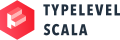Injection: Creating Custom Encoders

Injection lets us define encoders for types that do not have one by injecting A into an encodable type B. This is the definition of the injection typeclass:

trait Injection[A, B] extends Serializable {
def apply(a: A): B
def invert(b: B): A
}

Example

Let's define a simple case class:

case class Person(age: Int, birthday: java.util.Date)
val people = Seq(Person(42, new java.util.Date))
// people: Seq[Person] = List(Person(42, Wed Jan 26 22:40:48 UTC 2022))

And an instance of a TypedDataset:

val personDS = TypedDataset.create(people)
// error: could not find implicit value for parameter encoder: frameless.TypedEncoder[repl.MdocSession.App0.Person]
// val personDS = TypedDataset.create(people)
//                ^^^^^^^^^^^^^^^^^^^^^^^^^^^

Looks like we can't, a TypedEncoder instance of Person is not available, or more precisely for java.util.Date. But we can define a injection from java.util.Date to an encodable type, like Long:

import frameless._
implicit val dateToLongInjection = new Injection[java.util.Date, Long] {
def apply(d: java.util.Date): Long = d.getTime()
def invert(l: Long): java.util.Date = new java.util.Date(l)
}
// dateToLongInjection: AnyRef with Injection[java.util.Date, Long] = repl.MdocSession\$App0\$\$anon\$1@483eafa9

We can be less verbose using the Injection.apply function:

import frameless._
implicit val dateToLongInjection = Injection((_: java.util.Date).getTime(), new java.util.Date((_: Long)))
// dateToLongInjection: Injection[java.util.Date, Long] = frameless.Injection\$\$anon\$1@264f876b

Now we can create our TypedDataset:

val personDS = TypedDataset.create(people)
// personDS: TypedDataset[Person] = [age: int, birthday: bigint]

Another example

Let's define a sealed family:

sealed trait Gender
case object Male extends Gender
case object Female extends Gender
case object Other extends Gender

And a simple case class:

case class Person(age: Int, gender: Gender)
val people = Seq(Person(42, Male))
// people: Seq[Person] = List(Person(42, Male))

Again if we try to create a TypedDataset, we get a compilation error.

val personDS = TypedDataset.create(people)
// error: could not find implicit value for parameter encoder: frameless.TypedEncoder[repl.MdocSession.App1.Person]
// val personDS = TypedDataset.create(people)
//                ^^^^^^^^^^^^^^^^^^^^^^^^^^^

Let's define an injection instance for Gender:

import frameless._
implicit val genderToInt: Injection[Gender, Int] = Injection(
{
case Male   => 1
case Female => 2
case Other  => 3
},
{
case 1 => Male
case 2 => Female
case 3 => Other
})
// genderToInt: Injection[Gender, Int] = frameless.Injection\$\$anon\$1@5432ce75

And now we can create our TypedDataset:

val personDS = TypedDataset.create(people)
// personDS: TypedDataset[Person] = [age: int, gender: int]

Alternatively, an injection instance can be derived for sealed families such as Gender using the following import, import frameless.TypedEncoder.injections._. This will encode the data constructors as strings.

Known issue: An invalid injection instance will be derived if there are data constructors with the same name. For example, consider the following sealed family:

sealed trait Foo
object A { case object Bar extends Foo }
object B { case object Bar extends Foo }

A.Bar and B.Bar will both be encoded as "Bar" thereby breaking the law that invert(apply(x)) == x.